HOME / Applications / TEAM 24: Nonlinear Time-Transient Rotational Test Rig

# TEAM 24: Nonlinear Time-Transient Rotational Test Rig

Used Tools:## General description of the problem

This problem serves as a benchmark for 3D time transient electromagnetic simulation. A test rig with similar configuration of a Switched Reluctance Machine is made of solid medium-carbon steel and mounted in a nonmagnetic cage. This cage rotates about a stainless-steel shaft. The dimensions of the test rig are shown in Figure 1.  The thickness of both the stator and rotor is 25.4 mm.  Figure 2 shows the geometrical parameters of each pole coil. The rotor is tilted at an angle of 22 degrees . Figure 3 shows a 3D model of the simulated test rig build inside SOLIDWORKS.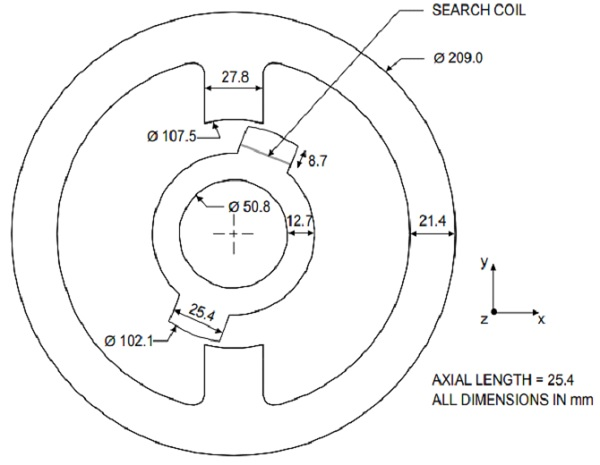Figure 1 -  Dimensions of stator and rotor.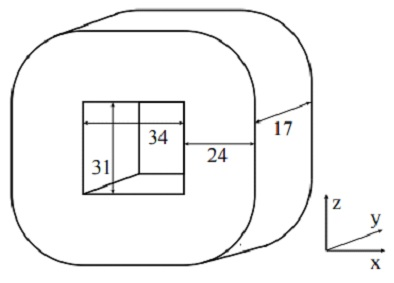Figure 2 - Dimensions of the coils.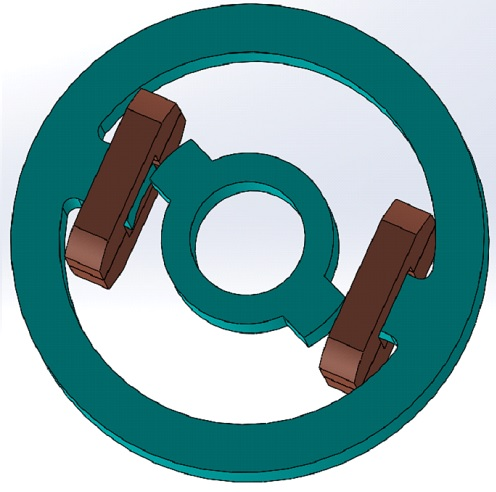Figure 3 - SOLIDWORKS model of the test rig.

## Time domain EM simulation using EMS for SOLIDWORKS

The Transient Magnetic module of EMS is used to compute and visualize magnetic fields that vary over time. These fields are typically caused by surges in currents or voltages. This type of analysis can be linear or non-linear. It also addresses eddy currents, power losses and magnetic forces.

To perform an analysis using EMS, the following steps are required :

• Create a new Transient Magnetic study
• Apply the right materials to all the parts
• Apply a suitable coil (either voltage or current driven) with the correct excitation.
• Run the simulation and look at the results

### Materials

After creating a transient magnetic study, the next step is to assign material to each component.

The stator and the rotor are made of EN9 steel. It is characterized by an electrical conductivity of 4.54 e+6 S/m and a BH curve shown in the Figure 4 below.  The coils are made of copper which has an electrical conductivity of 57.7e+6 S/m. Air is applied to all the other parts.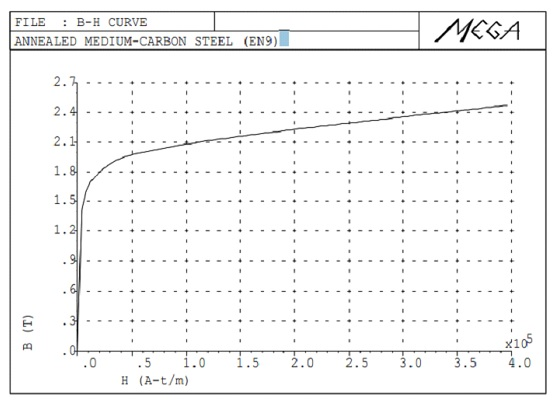Figure 4: Measured initial magnetization curve .

### Coil excitation

In this problem, 350-turns of copper wire is used to make the winding. Figure 5 shows the input current of each winding.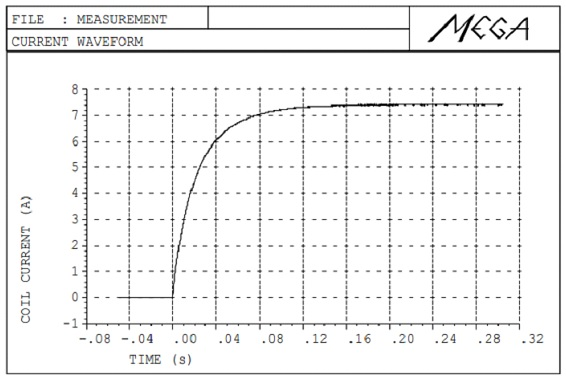Figure 5 - Input current for each winding.

To compute the magnetic force and torque produced on the rotor, a virtual work on the rotor is defined.

#### Mesh

Mesh quality is critical to every FEM simulation. The results accuracy and the solving time are strongly dependent on the mesh size. EMS allows user to control the mesh size on solid bodies and faces through its Mesh control feature. In this example, several mesh controls are applied as shown in Table 1. Figure 6 shows the meshed model.

Table 1: Applied mesh controls

 Bodies / components Mesh control size Coils and shaft 6 mm Stator and rotor 4 mm Air gaps 0.5 mm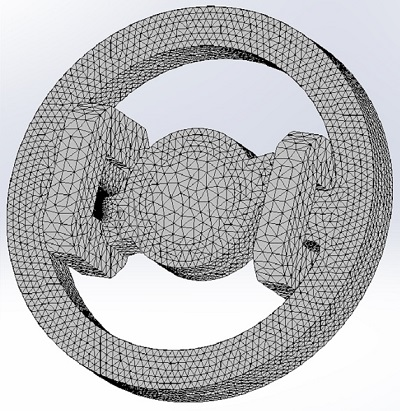Figure 6 -  Meshed model.

### EMS results

Once the solution is completed, the following results are created: magnetic flux density, magnetic field intensity, eddy current, inductance, impedance, flux linkage, current, induced voltage, force, torque and losses etc.

Figures 7 and 8 shows respectively fringe and vector plot of the magnetic flux density in the model at two times – 0.24 sec and 0.195 sec. The eddy current induced on both the stator and the rotor is plotted in Figure 9.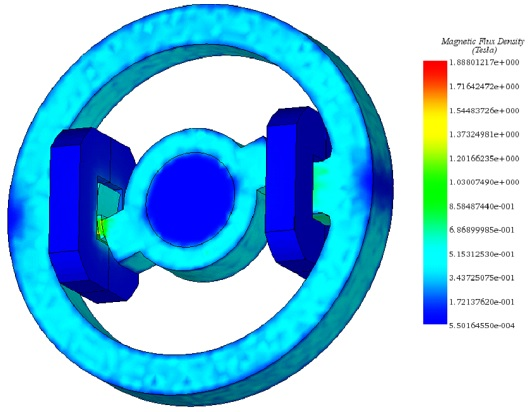Figure 7 - Fringe plot of the magnetic flux density at 0.24 s.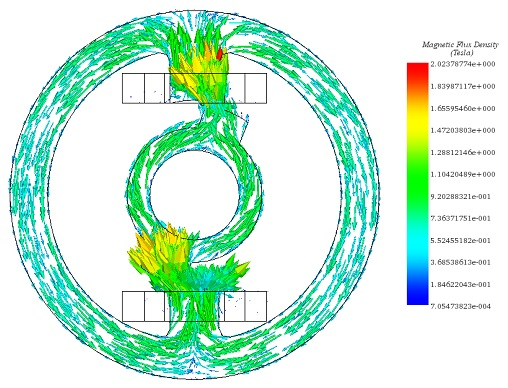Figure 8 - Vector plot of the magnetic flux density at 0.195 sec on the middle section of the model.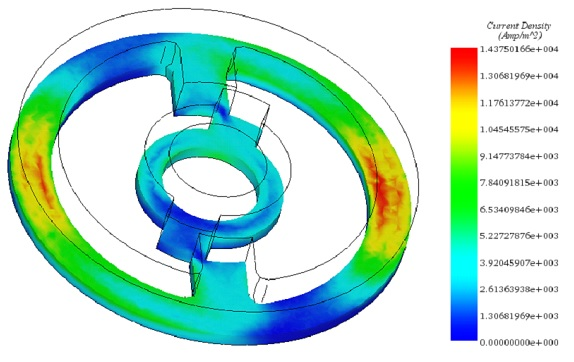Figure 9 -  Eddy current density at 0.17 sec.

Figure 10 shows a comparison of the torque variation between EMS and TEAM 24 results. We find from the plot that EMS result shows good agreement with the compared benchmark.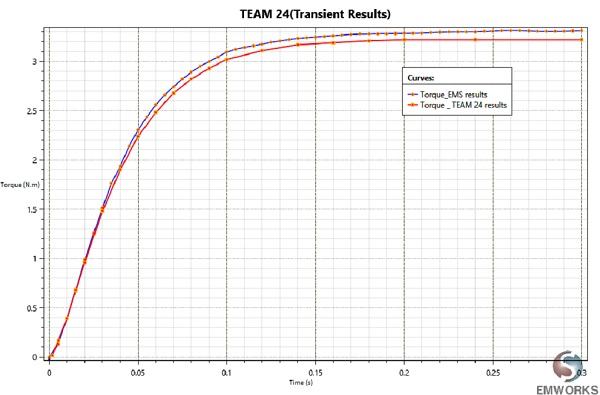Figure 10 - Torque result comparison.

## Conclusion

Electromagnetic transient problems could be simulated using the Transient Magnetic module of EMS. The results published in this note show excellent agreement with the benchmark. Transient magnetic solver can be coupled to both the Motion and the Thermal solver to study several applications such as motors, generators, eddy current braking systems, NDT sensors etc.

## References

 http://www.compumag.org/jsite/images/stories/TEAM/problem24.pdf